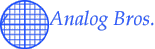Basic Electronics Help

Here's some stuff to help you figure out speaker cabinet total impedances, etc....

Ohm's Law

Ohm's Law describes the relationships among voltage, resistance, and current. The basic form of the equation is:

V = I / R

where V is voltage, I is current in Amps, and R is resistance in Ohms. If you know any two values, you can calculate the third. Click on this button to calculate for voltage, current, or resistance:
Once you know two of the above values, you can also calculate for Power in Watts:©1998-2006 Analog Bros.Bros.All Rights Reserved